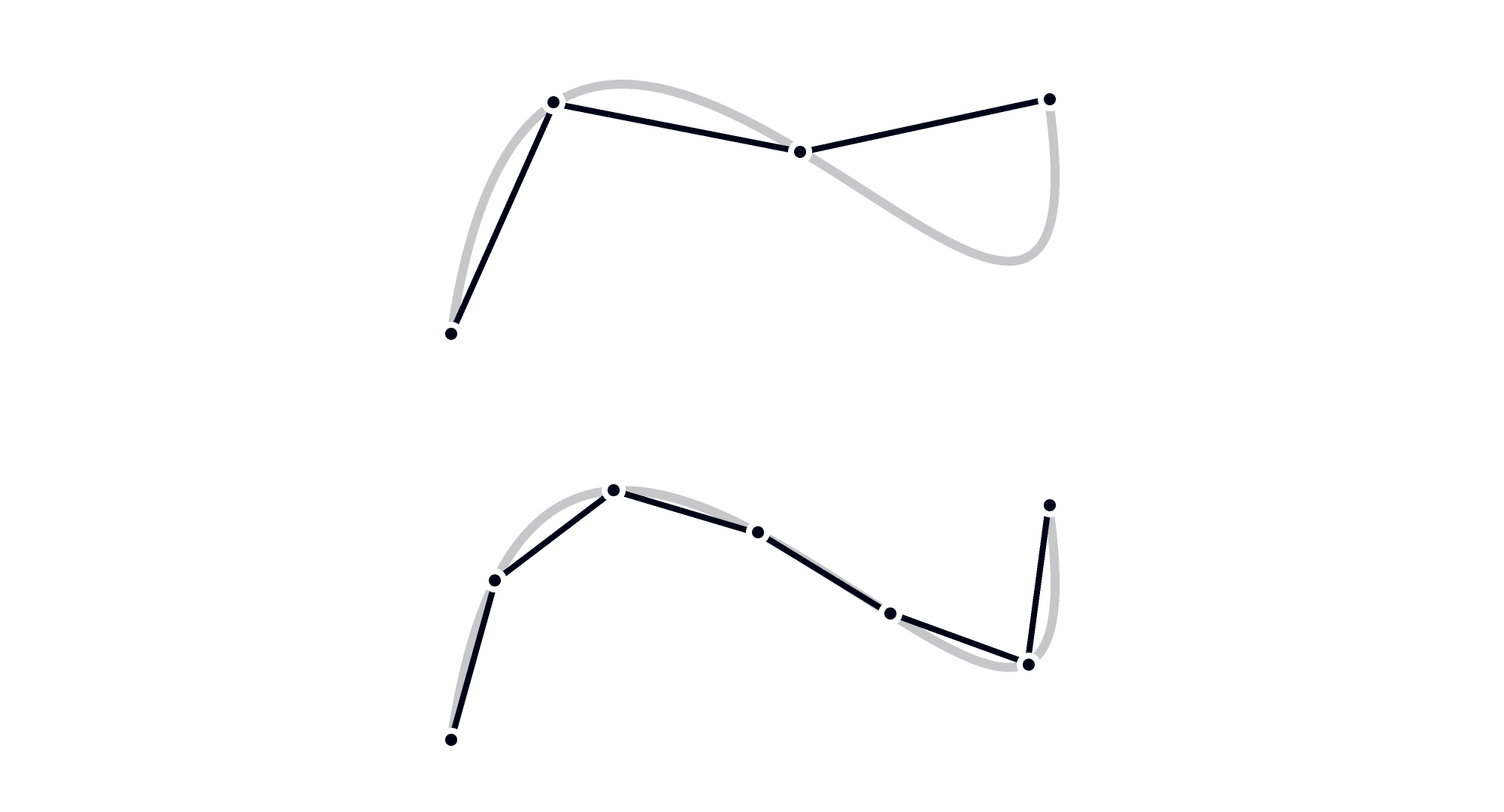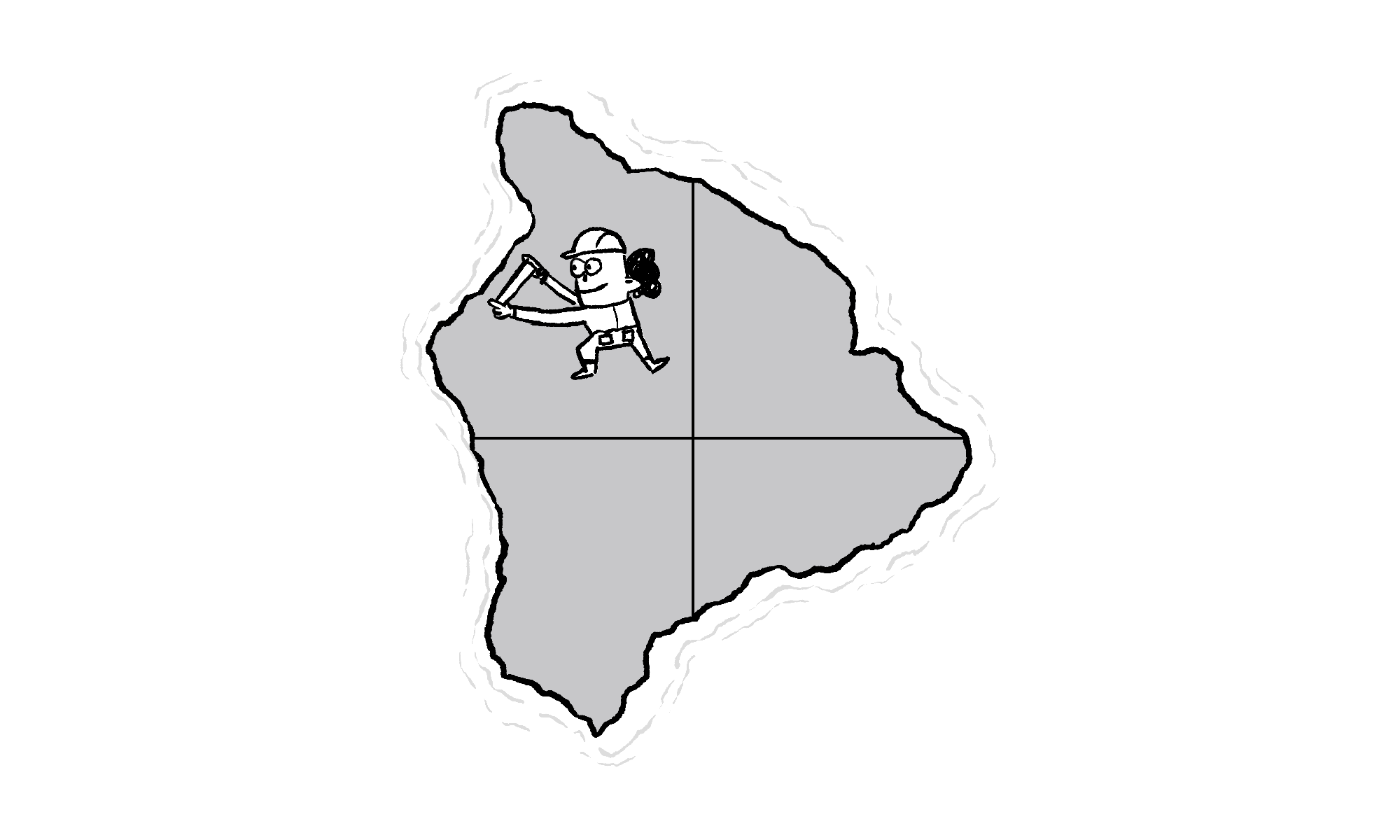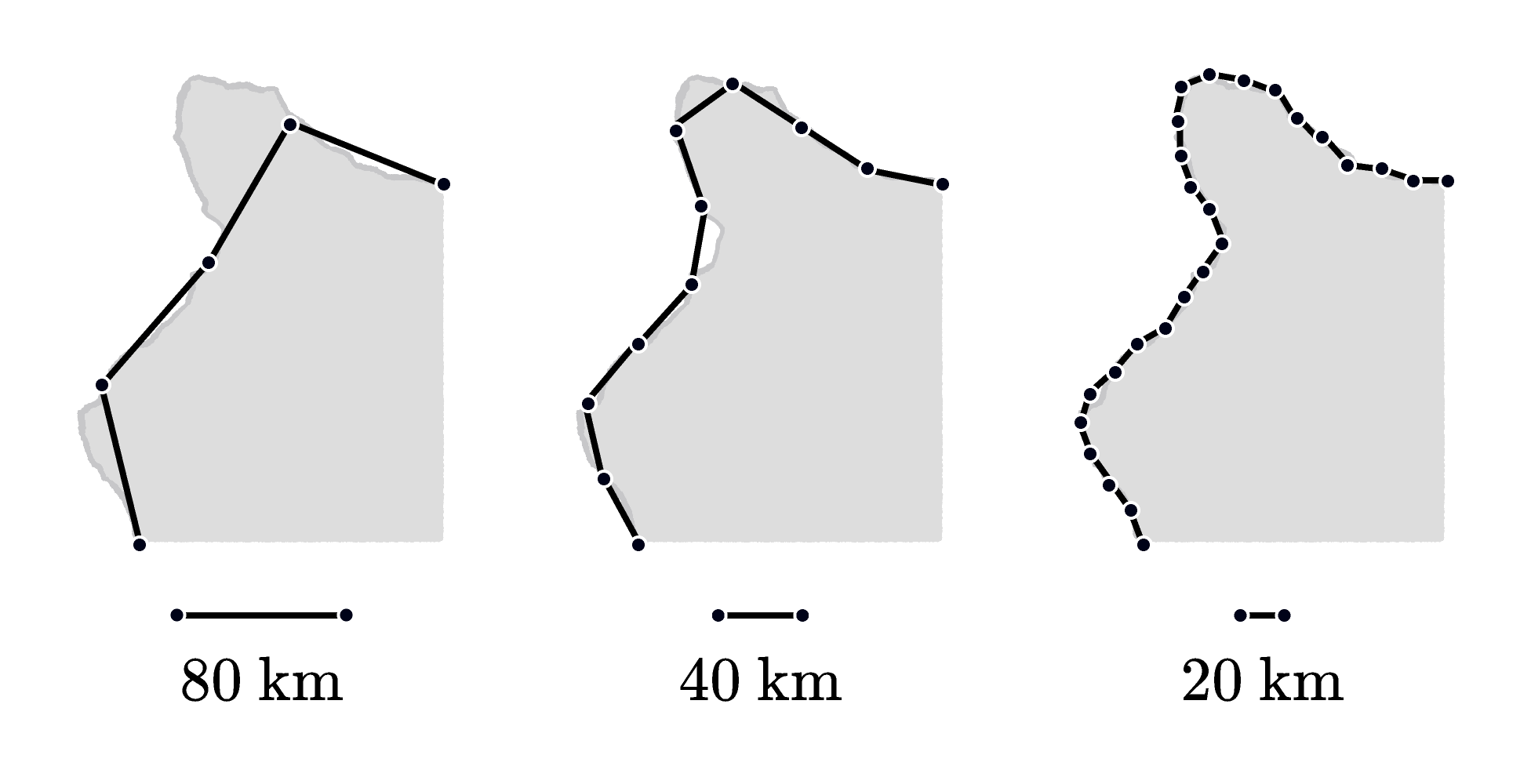## Measuring a Coastline

How long is a coastline? Depending on who you ask and how they measure, you could get wildly different answers. Even an infinite length is a plausible answer! This is known as the coastline paradox.

An understanding of dimension, as introduced in the previous challenge of this series, can shed some light on the coastline paradox. For a look at measuring curves and how that relates to dimension, keep reading. Or, jump straight to today’s coastline dimension challenge.

We can estimate the length of a smooth curve by a simple process: grab a ruler and estimate it with straight lines. As you use shorter and shorter rulers, your answer gets better and better. The reason this works well is that if you look at a small bit of a curved line, it’s actually pretty straight.Shorter lines fit closer to the curve.

But a jagged coastline isn’t like this. Even as we zoom in on a small section of it, it’s not that straight. When we look closer, more rough edges are revealed. So even as we use smaller and smaller rulers, the length of the coast doesn’t converge toward a fixed answer, it just keeps getting bigger and bigger.

Is the length of a coast infinite? Well, if the coast is jagged no matter how closely we look, then by the reasoning above, yes, it is infinite. In practice though, as you get to really, really small lengths, things tend to smooth out in the real world. However, since we don't usually measure coastlines with electron microscopes, the paradox is a real one, with real consequences for how we talk about the measurement of rough edged-surfaces.

When dealing with jagged surfaces like this, how can we quantify how jagged it is? That's where the idea of dimension comes in.

A smooth line is 1-dimensional ($D=1$). That's because if you measure it with a ruler that is two times shorter ($S=2$), you can fit in approximately two times as many of them ($C=2$). We can see that kind of scaling at work in this picture.Shorter lines fit closer to the curve.

This satisfies the equation we found for dimension in a previous daily challenge: $C = S^D.$

Using the same relationship: if a shape was two-dimensional $D=2,$ and you scaled down your measuring device by a factor of two, you'd expect to fit about $C=4$ times as many.What dimension is a jagged coastline? That's what you'll find out in this daily challenge.

# Today's ChallengeThe people of the nation Brilliantia are trying to find the length of their coastline. Unfortunately, their coast is jagged and uneven no matter how far you zoom in. They found that as they used shorter rulers, the length of the coastline seemed to increase from $320,$ to $400,$ to $500$ kilometers$).$

Here's what happened when they tried to measure the coast:

• An $80$-kilometer ruler fit along the coastline $4$ times.
• A $40$-kilometer ruler fit along the coastline $10$ times.
• A $20$-kilometer ruler fit along the coastline $25$ times.Every time they halved the size of their rulers, they expected to approximately double the number that fit along the coast. (This would be the case if the coast was a one-dimensional object like a smooth line.)

Since the coastline doesn't seem to be a one-dimensional object, what dimension does it have?

Hint: The dimension $D$ of an object satisfies the equation $C = S^D,$ where $S$ represents the amount of linear change (scale), and $C$ represents the number of copies of the original object we have after scaling.